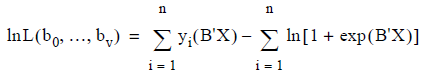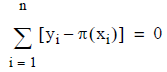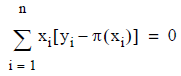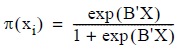# 5.4.5 - Maximum Likelihood - Teradata Warehouse Miner

## Teradata Warehouse Miner User Guide - Volume 3Analytic Functions

Product
Release Number
5.4.5
Published
February 2018
Language
English (United States)
Last Update
2018-05-04
dita:mapPath
yuy1504291362546.ditamap
dita:ditavalPath
ft:empty

In Linear Regression analysis, it is possible to use a least-squares approach to finding the best b- values in the linear regression equation. The least-squared error approach leads to a set of n normal equations in n unknowns that can be solved for directly. But that approach does not work here for logistic regression. Suppose any b- values are selected and the question is asked what is the likelihood that they match the logistic distribution defined, using statistical principles and the assumption that errors have a normal probability distribution. This technique of picking the most likely b- values that match the observed data is known as a maximum likelihood solution. In the case of linear regression, a maximum likelihood solution turns out to be mathematically equivalent to a least squares solution. But here maximum likelihood must be used directly.

For convenience, compute the natural logarithm of the likelihood function so that it is possible to convert the product of likelihood’s into a sum, which is easier to work with. The log likelihood equation for a given vector B of b-values with v x-variables is given by:where

B’X = b 0 + b 1 x 1 + ... + b v x v .

By differentiating this equation with respect to the constant term b 0 and with respect to the variable terms b i , the likelihood equations are derived:andwhereThe log likelihood equation is not linear in the unknown b- value parameters, so it must be solved using non-linear optimization techniques described in Computational Technique.